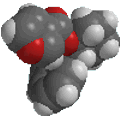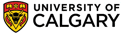﻿ Nomenclature rulesBasic IUPAC Organic Nomenclature

### Basic rules

The IUPAC systematic name of an organic compound can be constructed based on a series of steps and rules:

• Identification of the principal functional group and substituents
• Identification of the longest continuous chain containing the principal functional group.
• Assign locants (i.e. numbering) to the principal functional group and substituents.
 Misconception Alert! When assigning the numbers (i.e. the locants) while naming an organic compound there is NO rule based on summing the numbers.

The steps and rules are summarised below, more details are provided as the cases are encountered.

 Principal Functional group The principal functional group is used to define the class the compound belongs to e.g. an alcohol, ROH The principal functional group is the highest priority functional group. Functional group priority is discussed later. The principal functional group is usually given the lowest locant possible. When a group is named as a substituent, the priority order does not apply. Longest chain The longest continuous chain containing the principal functional group defines the root name.  Other groups attached to this chain are called substituents. If there are two chains of equal length, then the choice that gives the simplest substituents is chosen. Numbering (i.e. assigning locants) The numbers that define the positions of the principal functional group and substituents are called locants. Compounds are numbered from one end of the longest continuous chain. The locants are assigned such that the principal functional group gets the lowest possible locant. If this results in a "tie" then the first point of difference rule is applied so that the first time a difference in numbering occurs, then the method that gives the lower number at this first difference is used. In the event that there is no first point of difference then alphabetisation is used. Note that a locant can only be omitted if they are a. "1" and b. would be unambiguous (see later).©Dr. Ian Hunt, Department of Chemistry